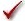## Please enable JavaScript to view this site.

 Navigation: PRINCIPLES OF STATISTICS > Analysis checklists Analysis checklist: Correlation

###Are the data points independent?

Correlation assumes that any random factor affects only one data point, and not others. You would violate this assumption if you choose half the subjects from one group and half from another. A difference between groups would affect half the subjects and not the other half.

###Are X and Y measured independently?

The calculations are not valid if X and Y are intertwined. You’d violate this assumption if you correlate midterm exam scores with overall course score, as the midterm score is one of the components of the overall score.

###Were X values measured (not controlled)?

If you controlled X values (e.g., concentration, dose, or time) you should calculate linear regression rather than correlation.

###Is the covariation linear?

A correlation analysis would not be helpful if Y increases as X increases up to a point, and then Y decreases as X increases further. You might obtain a low value of r, even though the two variables are strongly related. The correlation coefficient quantifies linear covariation only.

###Are X and Y distributed according to Gaussian distributions?

To accept the P value from standard (Pearson) correlation, the X and Y values must each be sampled from populations that follow Gaussian distributions. Spearman nonparametric correlation does not make this assumption.